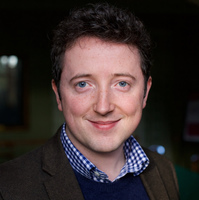# Computing disconnected bifurcation diagrams of partial differential equations#### Keywords

Speaker:
Patrick Farrell
Affiliation:
Mathematical Institute, Oxford
When:
Thursday 26th April 2018
Time:
14:00:00
Where:
Aula Consiglio VII Piano - Edificio 14, Dipartimento di Matematica POLITECNICO DI MILANO
Abstract:
Computing the distinct solutions $u$ of an equation $f(u, \lambda) = 0$ as a parameter $\lambda \in \mathbb{R}$ is varied is a central task in applied mathematics and engineering. The solutions are captured in a bifurcation diagram, plotting (some functional of) $u$ as a function of $\lambda$. In this talk I will present a new algorithm, deflated continuation, for this task. Deflated continuation has three advantages. First, it is capable of computing disconnected bifurcation diagrams; previous algorithms only aimed to compute that part of the bifurcation diagram continuously connected to the initial data. Second, its implementation is extremely simple: it only requires a minor modification to any existing Newton-based solver. Third, it can scale to very large discretisations if a good preconditioner is available. Among other problems, we will apply this to a famous singularly perturbed ODE, Carrier's problem. The computations reveal a striking and beautiful bifurcation diagram, with an infinite sequence of alternating pitchfork and fold bifurcations as the singular perturbation parameter tends to zero. The analysis yields a novel and complete taxonomy of the solutions to the problem. We will also apply it to discover previously unknown solutions to equations arising in liquid crystals and quantum mechanics. This seminar is organized within the Research project MFAG 17412 «Mathematical insights of glioblastoma growth: a mechano-biology approach for patientspecific clinical tools» funded by the Italian Association for Cancer Research (AIRC), Project coordinator Prof. Pasquale Ciarletta Contact: pasquale.ciarletta@polimi.it
Note:
I am an Associate Professor of Numerical Analysis and Scientific Computing and EPSRC Early Career Research Fellow in the Numerical Analysis group of the University of Oxford, and a Tutorial Fellow at Oriel College, Oxford. I work on the numerical solution of partial differential equations, with a particular focus on bifurcation analysis of nonlinear equations, the automated derivation and application of adjoint models, and the interaction between computational geometry and numerical simulation. I apply the numerical techniques I develop to various applications, including tidal turbines for renewable energy, bidomain cardiac electrophysiology, radiation transport, and glaciology. I mainly code in Python, contribute regularly to the FEniCS and PETSc software projects, and lead the development of dolfin-adjoint.